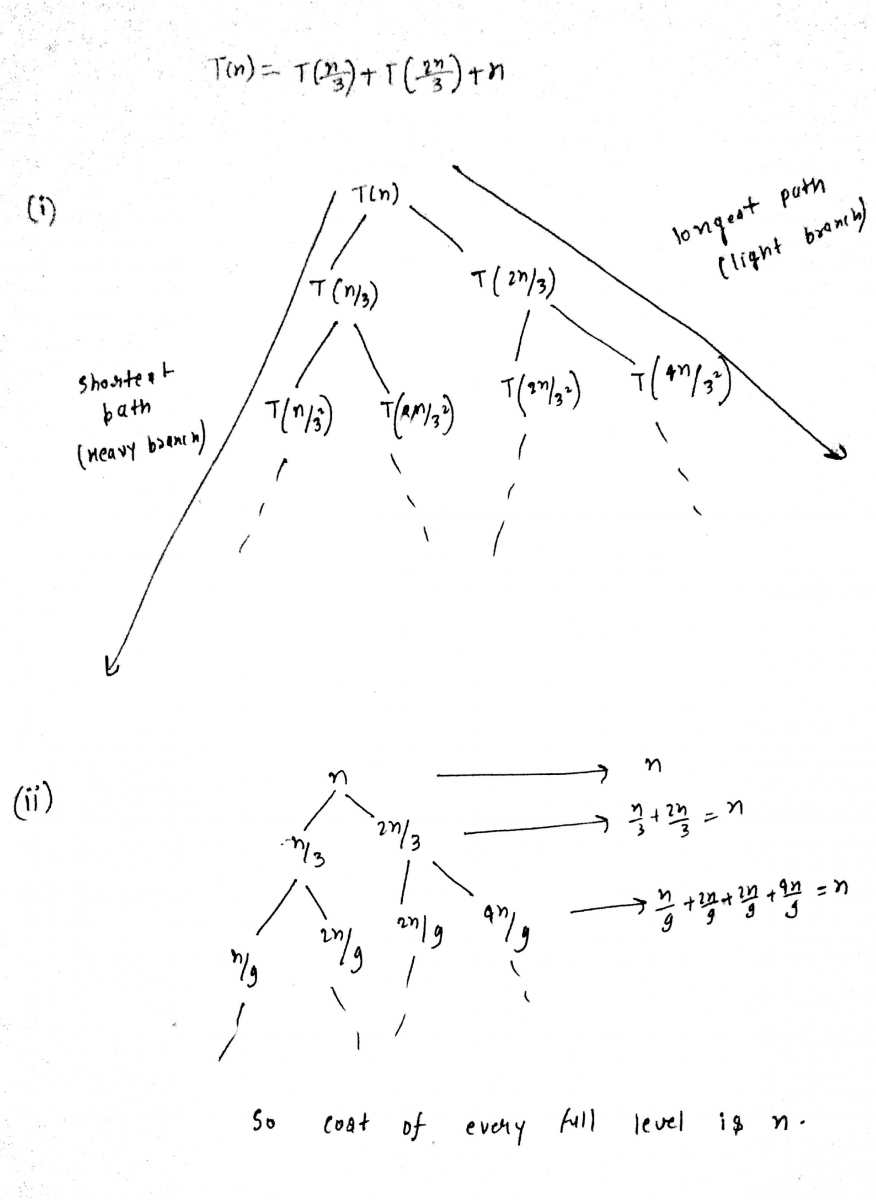##### How to find time complexity of such function which is not in form of masters Theorem?

How to find time complexity of such function which is not in form of masters Theorem?

T(N/3) + T(2N/3) + N

Sumit Verma
24 Apr 2017 12:29 am
Abhishek
24 Apr 2017 12:29 pm

Not able to understand.......

Sumit Verma
24 Apr 2017 01:27 pm

@abhisinu
See the recursion tree below,In the (i) recursion tree,
The shortest path to a leaf occurs when we take the heavy branch each time. The height k is given by n(1\3)k<=1 meaning n<= 3k or k>=log3n.
The longest path to a leaf occurs when we take the light branch each time.The height k is given by n(2\3)k<=1 meaning n<= (3/2)k or k>=log3/2n.
So if we want to get the lower bound, we will take the shortest path. You know that there are k levels and cost at every level is n. (See 2nd recursion tree).
Hence total cost = n * k = n * log3
Time complexity = omege (n log3n).
Please let me know if you still have any doubt.

Abhishek
24 Apr 2017 04:44 pm

what is heavy and lighter branch?

I understand overall but doubt in heavy and lighter branch.

Thanks for best explaination

Sumit Verma
24 Apr 2017 05:05 pm

Heavy branch means the branching is going to end soon. Here it is becoming 1/3rd after every branch.
Lighter branch means the branching is not going to end soon. Here it becoming 2/3rd after every branch.(Lighter in comparison to the first one)